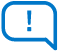Feedback
If you are having issues with the exercises, please create a ticket on DevZone: devzone.nordicsemi.com
Click or drag files to this area to upload. You can upload up to 2 files.# Exercise 2

## Mutexes

In this exercise, we will create an application with two threads running and accessing the same code section of code. The logic looks perfect when only one thread is accessing the critical section, but when two different threads try to access the code section simultaneously, unexpected things happen(Race condition). We will see how a mutex can be utilized to synchronize these two threads.

The threads we will create here will have the same priority (4), and they will have time slicing enabled for a period of 10 ms. This means each thread will be given 10 ms to finish its task before it’s forcefully preempted by the scheduler to allow the other equal priority threads to run.

### Exercise steps

1. In the GitHub repository for this course, open the base code for this exercise, found in `lesson8/fund_less8_exer2` of whichever version directory you are using (`v2.x.x` or `v1.6.0-v1.9.1`).

2. Enable multithreading in the application. This is done by adding the following in `prj.conf`:

``CONFIG_MULTITHREADING=y``

This configuration defaults to yes in all nRF Connect SDK applications and isn’t strictly necessary to enable manually.

3. Set the priority of thread0 and thread1 to have equal priority.

``````#define THREAD0_PRIORITY        4

4. Create the functions for the two threads. The functions should run the function `shared_code_section()` in a while-loop. `shared_code_section()` should be commented out in thread1 for now as shown below.

``````void thread0(void)
{
while (1) {
shared_code_section();
}
}

{
while (1) {
//shared_code_section();
}
}``````

5. Define two counter variables, `increment_count `and `decrement_count` and a constant macro `COMBINED_TOTAL `to set their combined total to an arbitrary value, let’s say 40.

``````#define COMBINED_TOTAL   40
int32_t increment_count = 0;
int32_t decrement_count = COMBINED_TOTAL; ``````

6. In the function shared_code_section(), add the following logic:

`increment_count `is incremented
`increment_count `is reset to 0 if the maximum of `COMBINED_TOTAL` is reached
`decrement_count `is decremented
`decrement_count `is reset to `COMBINED_TOTAL` if the minimum value of 1 is reached

``````increment_count += 1;
increment_count = increment_count % COMBINED_TOTAL;
decrement_count -= 1;
if (decrement_count == 0)
{
decrement_count = COMBINED_TOTAL;
}``````

#### Note

If this logic is implemented correctly `increment_count ``decrement_count ``COMBINED_TOTAL` should be true at all times. If this hard rule is broken at any point in the run time, there is a flaw in the code(race condition).

7. Add an if-statement in `shared_code_section()` that prints the values of the counters only if their combined value is not equal to `COMBINED_TOTAL`.

``````	if(increment_count + decrement_count != COMBINED_TOTAL )
{
printk("Race condition happend!\n");
printk("Increment_count (%d) + Decrement_count (%d) = %d \n",
increment_count, decrement_count, (increment_count + decrement_count));
k_msleep(400 + sys_rand32_get() % 10);
}``````

8. Build the application and flash it on your development kit. Using a serial terminal you should see the below output:

#### Note

The two threads are given a start-up scheduling delay of 5000 milliseconds, which only affects the first time the thread is put in the ready state. The reason why this dealy was added is to give you enough time to connect to the serial terminal so that you can observe the logging output.
``````*** Booting Zephyr OS build v3.2.99-ncs2 ***
``````

We confirm that our increment and decrement counter logic is working fine (in sequence) when only thread0 is accessing `shared_code_section()`, because the `printk() `check isn’t printing anything.

9. Now let `thread1` access `shared_code_section()` by uncommenting the line to make it look like below.

``````void thread1(void)
{
while (1) {
shared_code_section();
}
}``````

10. Build the application and flash it on your development kit again. You should see the below output:

``````
*** Booting Zephyr OS build v3.2.99-ncs2 ***
Race condition happend!
Increment_count (6) + Decrement_count (35) = 41
Race condition happend!
Increment_count (7) + Decrement_count (34) = 41
Race condition happend!
Increment_count (8) + Decrement_count (33) = 41
Race condition happend!
Increment_count (0) + Decrement_count (1) = 1
Race condition happend!
Increment_count (10) + Decrement_count (31) = 41
Race condition happend!
Increment_count (11) + Decrement_count (30) = 41
Race condition happend!
Increment_count (12) + Decrement_count (29) = 41
Race condition happend!
Increment_count (13) + Decrement_count (28) = 41
Race condition happend!
Increment_count (14) + Decrement_count (27) = 41
Race condition happend!
Increment_count (15) + Decrement_count (26) = 41
Race condition happend!
Increment_count (16) + Decrement_count (25) = 41
Race condition happend!
Increment_count (17) + Decrement_count (24) = 41 ``````

As soon as `thread1` started to access the shared code section, our working logic failed.

This is a classic example for the need to protect the shared code section to ensure that only one thread can access the shared code section (critical section) at one time. The basic flaw in our code is that we assume that the operations on the two counters (`increment_count`, `decrement_count`) will be performed at once without interruptions. When this is not the case, because any thread can access and update these shared memory variables anytime, then the checks on the values will fail. This is where we can use a mutex to serialize the access to this shared code by multiple threads. By using a mutex, we can force `shared_code_section()` to run uninterrupted by other threads.

11. Add a mutex by first defining the mutex like below.

``K_MUTEX_DEFINE(test_mutex);``

12.1 Lock the mutex before performing the counter logic

``k_mutex_lock(&test_mutex, K_FOREVER);``

12.2 Unlock it right before the if-statement that checks the counter sum using these functions.

``k_mutex_unlock(&test_mutex);``

13. Build the application and flash it on your development kit. You should see the same output as in step 8, i.e the values of the counters are not printed.

The solution for this exercise can be found in the GitHub repository, `lesson7/fund_less8_exer2_solution` of whichever version directory you are using (`v2.x.x` or `v1.6.0-v1.9.1`).

Register an account